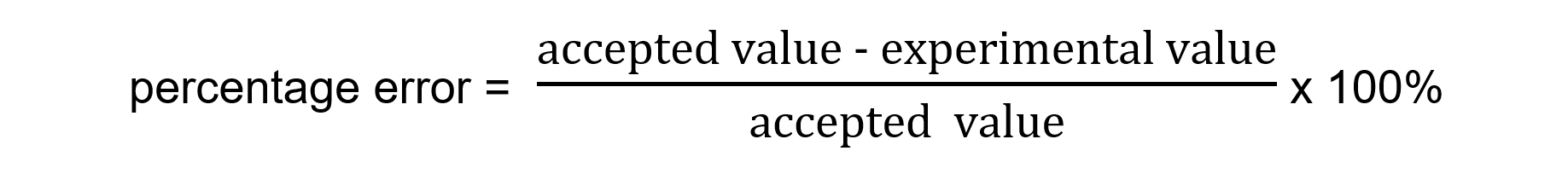# IB DP Chemistry: SL复习笔记11.2.4 Percentage Error

### Percentage Error

• Percentage error is used to express the difference between a final calculated answer and an accepted or literature value
• It is calculated using the following formula• You should be able to comment on any differences between the experimental and literature values

#### Worked Example

1.023 g of propan-1-ol (M = 60.11 g mol-1) was burned in a spirit burner and used to heat 200 g of water in a copper calorimeter. The temperature of the water rose by 30 oC.

1. Calculate the enthalpy of combustion of propan-1-ol using this data.
2. The literature value for this enthalpy change is -2021 kJ mol-1. Calculate the percentage error and comment on your findings

Step 1: Calculate q

q = m x c x ΔT

q = 200 g x 4.18 J g-1 K-1 x 30 K = – 25 080 J

Step 2: Calculate the amount of propan-1-ol burned

moles = mass ÷ molar mass = 1.023 g ÷  60.11 g mol-1 = 0.01702 mol

Step 3: Calculate ΔH

ΔH = q ÷ n =  -25 080 J ÷ 0.01702 mol = – 1 473 560 J = -1 474 kJ = -1.5 x 103 kJ

Using the formulaHeat losses are likely to be the largest source of error in this experiment